# Python | Implementation of Movie Recommender System

Recommender System is a system that seeks to predict or filter preferences according to the user’s choices. Recommender systems are utilized in a variety of areas including movies, music, news, books, research articles, search queries, social tags, and products in general.

Recommender systems produce a list of recommendations in any of the two ways –

• Collaborative filtering: Collaborative filtering approaches build a model from user’s past behavior (i.e. items purchased or searched by the user) as well as similar decisions made by other users. This model is then used to predict items (or ratings for items) that user may have an interest in.
• Content-based filtering: Content-based filtering approaches uses a series of discrete characteristics of an item in order to recommend additional items with similar properties. Content-based filtering methods are totally based on a description of the item and a profile of the user’s preferences. It recommends items based on user’s past preferences.

Let’s develop a basic recommendation system using Python and Pandas.

Let’s focus on providing a basic recommendation system by suggesting items that are most similar to a particular item, in this case, movies. It just tells what movies/items are most similar to user’s movie choice.

Import dataset with delimiter “\t” as the file is a tsv file (tab separated file).

 `# import pandas library ` `import` `pandas as pd ` ` `  `# Get the data ` `column_names ``=` `[``'user_id'``, ``'item_id'``, ``'rating'``, ``'timestamp'``] ` ` `  `path ``=` `'https://media.geeksforgeeks.org/wp-content/uploads/file.tsv'` ` `  `df ``=` `pd.read_csv(path, sep``=``'\t'``, names``=``column_names) ` ` `  `# Check the head of the data ` `df.head() `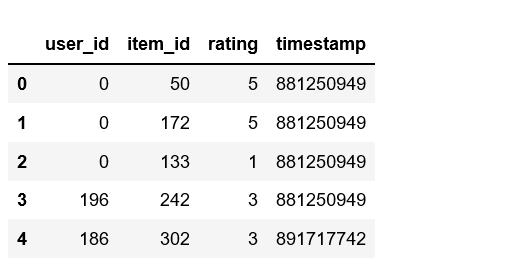`# Check out all the movies and their respective IDs ` `movie_titles ``=` `pd.read_csv(``'https://media.geeksforgeeks.org/wp-content/uploads/Movie_Id_Titles.csv'``) ` `movie_titles.head() `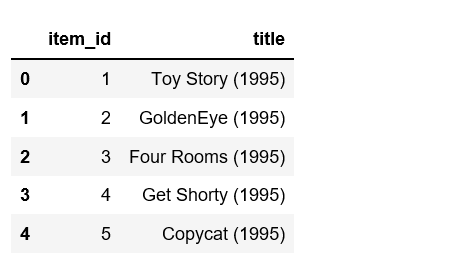`data ``=` `pd.merge(df, movie_titles, on``=``'item_id'``) ` `data.head() `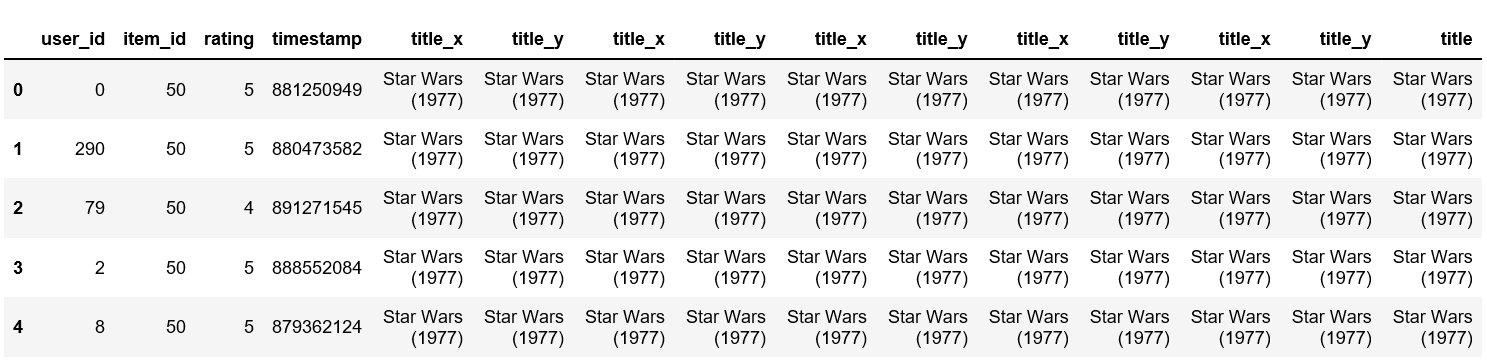`# Calculate mean rating of all movies ` `data.groupby(``'title'``)[``'rating'``].mean().sort_values(ascending``=``False``).head() `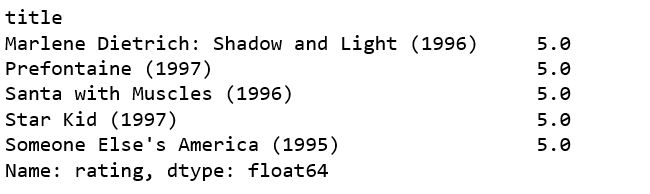`# Calculate count rating of all movies ` `data.groupby(``'title'``)[``'rating'``].count().sort_values(ascending``=``False``).head() `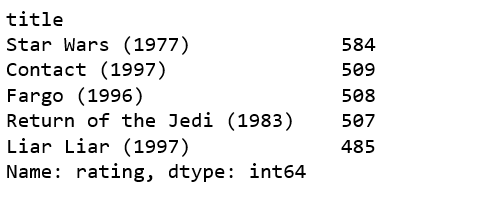`# creating dataframe with 'rating' count values ` `ratings ``=` `pd.DataFrame(data.groupby(``'title'``)[``'rating'``].mean())  ` ` `  `ratings[``'num of ratings'``] ``=` `pd.DataFrame(data.groupby(``'title'``)[``'rating'``].count()) ` ` `  `ratings.head() `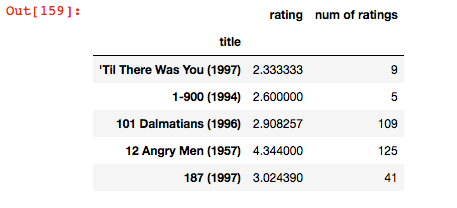Visualization imports:

 `import` `matplotlib.pyplot as plt ` `import` `seaborn as sns ` ` `  `sns.set_style(``'white'``) ` `%``matplotlib inline `

 `# plot graph of 'num of ratings column' ` `plt.figure(figsize ``=``(``10``, ``4``)) ` ` `  `ratings[``'num of ratings'``].hist(bins ``=` `70``) `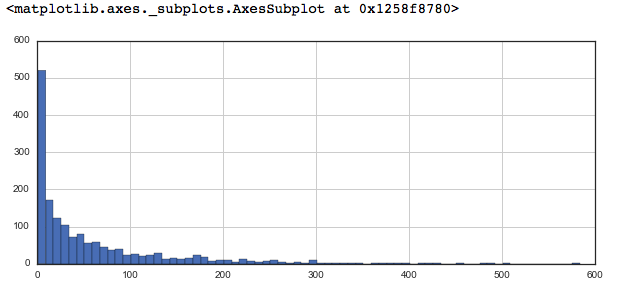`# plot graph of 'ratings' column ` `plt.figure(figsize ``=``(``10``, ``4``)) ` ` `  `ratings[``'rating'``].hist(bins ``=` `70``) `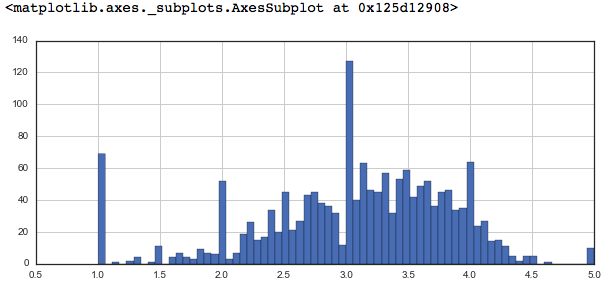`# Sorting values according to  ` `# the 'num of rating column' ` `moviemat ``=` `data.pivot_table(index ``=``'user_id'``, ` `              ``columns ``=``'title'``, values ``=``'rating'``) ` ` `  `moviemat.head() ` ` `  `ratings.sort_values(``'num of ratings'``, ascending ``=` `False``).head(``10``) `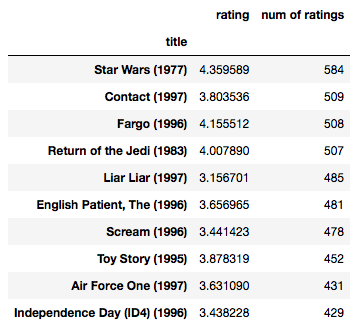`# analysing correlation with similar movies ` `starwars_user_ratings ``=` `moviemat[``'Star Wars (1977)'``] ` `liarliar_user_ratings ``=` `moviemat[``'Liar Liar (1997)'``] ` ` `  `starwars_user_ratings.head() `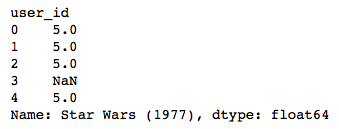`# analysing correlation with similar movies ` `similar_to_starwars ``=` `moviemat.corrwith(starwars_user_ratings) ` `similar_to_liarliar ``=` `moviemat.corrwith(liarliar_user_ratings) ` ` `  `corr_starwars ``=` `pd.DataFrame(similar_to_starwars, columns ``=``[``'Correlation'``]) ` `corr_starwars.dropna(inplace ``=` `True``) ` ` `  `corr_starwars.head() `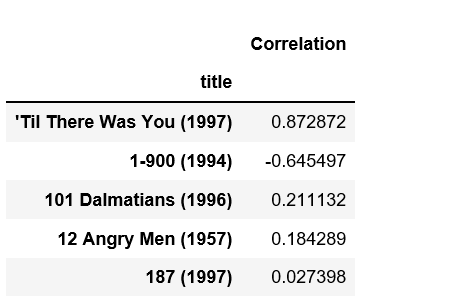`# Similar movies like starwars ` `corr_starwars.sort_values(``'Correlation'``, ascending ``=` `False``).head(``10``) ` `corr_starwars ``=` `corr_starwars.join(ratings[``'num of ratings'``]) ` ` `  `corr_starwars.head() ` ` `  `corr_starwars[corr_starwars[``'num of ratings'``]>``100``].sort_values(``'Correlation'``, ascending ``=` `False``).head() `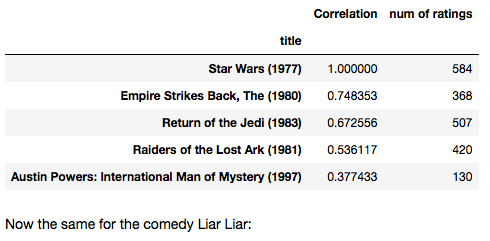`# Similar movies as of liarliar ` `corr_liarliar ``=` `pd.DataFrame(similar_to_liarliar, columns ``=``[``'Correlation'``]) ` `corr_liarliar.dropna(inplace ``=` `True``) ` ` `  `corr_liarliar ``=` `corr_liarliar.join(ratings[``'num of ratings'``]) ` `corr_liarliar[corr_liarliar[``'num of ratings'``]>``100``].sort_values(``'Correlation'``, ascending ``=` `False``).head() `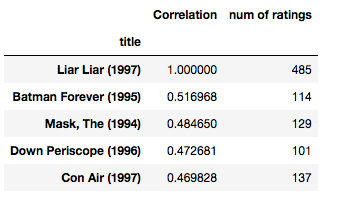Attention geek! Strengthen your foundations with the Python Programming Foundation Course and learn the basics.

To begin with, your interview preparations Enhance your Data Structures concepts with the Python DS Course.

My Personal Notes arrow_drop_upCheck out this Author's contributed articles.

If you like GeeksforGeeks and would like to contribute, you can also write an article using contribute.geeksforgeeks.org or mail your article to contribute@geeksforgeeks.org. See your article appearing on the GeeksforGeeks main page and help other Geeks.

Please Improve this article if you find anything incorrect by clicking on the "Improve Article" button below.